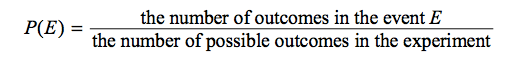In this section, the following topics are discussed with examples

• Introduction
• Probability Terminologies
• Probability Facts
• Examples

### Introduction

Probability is a way of describing uncertainty in numerical terms. In this section we review some of the terminology used in elementary probability theory.

### Understanding of terminologies of Probability:

If any two events have no common outcomes then that event is called Mutually exclusive event.

For example: While tossing a coin , if heads is on the face then the tails wont happen this is mutually exclusive event .

If suppose happening of one event doesn’t affect the other then it is Independent event

For example: While tossing two coins , heads of one coin won’t affect other coin’s outcome.

Two events are said to dependent if happening of one event affects the happening of other or the next event.

For example: While drawing a card from the pack of 52 cards , when one card is take out and if without replacement if we take the next card then the second outcome is the dependent of first outcome.

If suppose we replace it , then the first and second outcomes are independent event.### General Facts

The following are general facts about probability.
• If an event is certain to occur, then P(E) = 1.

• If an event is certain not to occur, then P(E) = 0.

• If an event is possible but not certain to occur, then 0 < P(E) < 1.

• The probability that an event will not occur is equal to 1 – P(E).

• If is an event, then the probability of is the sum of the probabilities of the outcomes in E. • The sum of the probabilities of all possible outcomes of an experiment is 1.

If and are two events of an experiment, we consider two other events related to and F. • The event that both and occur, that is, all outcomes in the set « F.
• The event that or F, or both, occur, that is, all outcomes in the set » F

Events that cannot occur at the same time are said to be mutually exclusive. For example, if a 6-sided die is rolled once, the event of rolling an odd number and the event of rolling an even number are mutually exclusive. But rolling a 4 and rolling an even number are not mutually exclusive, since 4 is an outcome that is common to both events.

For events and F, we have the following rules.
• P(or F, or both, occur) = P(E) + P(F) – P(both and occur), which is the inclusion-

exclusion principle applied to probability.
• If and are mutually exclusive, then P(both and occur) = 0, and therefore,

P(or F, or both, occur) = P(E) + P(F).

• and are said to be independent if the occurrence of either event does not affect the occurrence of the other. If two events and are independent, then P(both and occur) = P(E)P(F).

## We must become more comfortable with probability and uncertainty.

Nate Silver

#### Example 1:

What’s the probability of getting heads when flipping a coin?

Solution:

There is only one way to get heads in a coin toss. Hence, the top of the probability fraction is 1. There are two possible results: heads or tails. Forming the probability fraction gives 1/2.

#### Example 2:

What’s the probability of getting a king when drawing a card from a deck of cards?

Solution:

A deck of cards has four kings, so there are 4 ways to get a king. Hence, the top of the fraction is 4. There are 52 total cards in a deck. Forming the probability fraction gives 4/52, which reduces to 1/13. Hence, there is 1 chance in 13 of getting a king.

#### Example 3:

A die is rolled and a coin is tossed, find the probability that the die shows an odd number and the coin shows a head.

Sol:

The sample space S of the experiment described in question 5 is as follows

S = { (1,H),(2,H),(3,H),(4,H),(5,H),(6,H)
(1,T),(2,T),(3,T),(4,T),(5,T),(6,T)}

Let E be the event “the die shows an odd number and the coin shows a head”. Event E may be described as follows

E={(1,H),(3,H),(5,H)}

The probability P(E) is given by
P(E) = n(E) / n(S) = 3 / 12 = 1 / 4 .